# DAV Class 3 Maths Chapter 9 Worksheet 3 Solutions

The DAV Class 3 Maths Solutions and DAV Class 3 Maths Chapter 9 Worksheet 3 Solutions of Weight offer comprehensive answers to textbook questions.

## DAV Class 3 Maths Ch 9 WS 3 Solutions

Question 1.
Ms. Neena bought 9 kg 750 g of sugar in the beginning of a month. She used 4 kg 500g of sugar during that month. How much sugar was left?
Solution:
The weight of sugar bought = 9 kg 750 g
Sugar used = 4 kg 500 g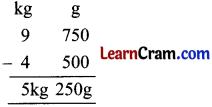5 kg 250 g sugar was left.

Question 2.
Mr. Rahul bought 8 kg 350 g of apples. He found that 1 kg 240 g of apples were unripe. Find the weight of ripe apples.
Solution:
The weight of apples bought = 8 kg 350 g
Unripe apples = 1 kg 240 gThe weight of ripe apples = 7 kg 110 g
7 kg 110 g of apples were ripe.Question 3.
A girl weighs 33 kg 520 g. She lost 1 kg 210 g due to illness. What is her weight now?
Solution:
The weight of girl = 33 kg 520 g
weight lost = 1 kg 210 g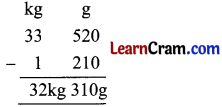Now her weight is 32kg 310 g

Question 4.
A housewife bought 2 kg 350 g potatoes and 1 kg 100 g tomatoes. Find the total weight of vegetables she bought.
Solution:
The weight of potatoes = 2 kg 350 g
The weight of tomatoes = 1 kg 100 g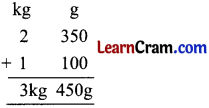Total weight of vegetables = 3 kg 450 g
Total weight of vegetables is 3 kg 450 g.### DAV Class 3 Maths Chapter 9 Value Based Questions

Ankur went to market ………………… and blessed Ankur.

Question 1.
Find the weight of the other bag and the old man was carrying.
Solution:

Question 2.
How can you offer help to old people?
Solution:

Total weight of bag = 6 kg 500 g
Weight Ankur took = 2 kg 750 g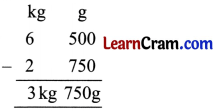Weight of other bag = 3 kg 750gQuestion 1.
Choose the correct weight to balance the side from given weight.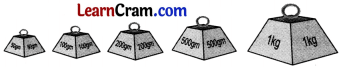(a)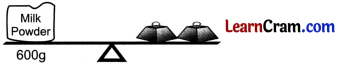Solution: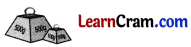(b)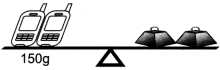Solution:(c)Solution: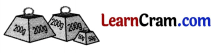Question 2.
Convert the following into grams:

(i) 3 kg 450 g
Solution:
3 kg 450 g = (3 × 1000 + 450)g
= (3000 + 450) g
= 3450 g.

(ii) 9 kg
Solution:
9 kg = 9 × 1000 g
= 9000 g

(iii) 30 kg 608 g
Solution:
30 kg 608 g = (30 × 1000) g + 608 g
=(30000 + 608)g
= 30608 g

(iv) 70 kg 50 g
Solution:
70 kg 50 g = (70 × 1000) g + 50 g
=(70000 + 50) g
= 70050 g

(v) 20 kg 150 g
Solution:
20kg 150g = (20 × 1000 + 150) g
= 20000 + 150g
= 20150 gQuestion 3.

(i)Solution:
87 kg 846 g

(ii)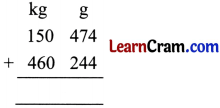Solution:
610 kg 718 g

(iii) 128g + 352g + 257g
Solution: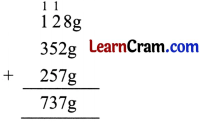Question 4.
Subtract

(i)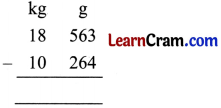Solution:
8 kg 299 gm

(ii)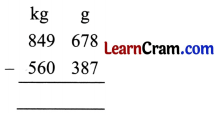Solution:
289 kg 291 g(iii) 8 kg 750 g – 3 kg 26 g
Solution:(iv) 90 kg 473 g – 61 kg 160 g
Solution: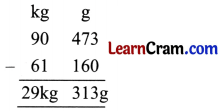Question 5.
Weight of Saurav is 5 kg 500 g more than the weight of Sita. If Sita’s weight is 70 kg 200 g find the weight of Saurav.
Solution:
Weight of Sita = 70 kg 200 g
The weight of Saurav is 5 kg 500g more than Sita
Weight of Saurav = 70 kg 200 g + 5 kg 500 gweight of Saurav = 75 kg 700 g### DAV Class 3 Maths Chapter 9 Worksheet 3 Notes

Word Problem

Question 1.
The weight of a box of toffees is 7 kg 260 g. The weight of the empty box is 1.960 kg. Find the weight of toffees only.
Solution:
The weight of box with toffees = 7 kg 260 g
The weight of empty box = 1 kg 960 g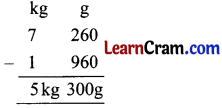The weight of toffees only = 5 kg 300 g